﻿ 小数据集的微地震信号震相拾取方法

# 小数据集的微地震信号震相拾取方法Phase Arrivals Picking Method for Microseismic Signals with Small Dataset

Abstract: In recent years, deep convolutional neural network has been extensively studied in the study of seismic phase pickup of seismic signals. However, such a model can pick up both P wave and S wave seismic phases, and it is well applied and popularized in seismic phase pickup of seismic data of natural seismic network. However, due to the large amount of tag data and high computational complexity, the application of such a model in microseismic monitoring with few tag samples is severely limited. Previous studies have shown that the complexity of P wave pickup is lower than that of S wave pickup. Only when P wave shock phase is picked up, the model complexity can be simpler and the label demand is lower. After the p-wave pickup is completed, the S-wave signature can be well extracted through polarization analysis and rotation, thus reducing the complexity of s-wave pickup. Based on this idea, this paper combines machine learning, polarization analysis, time-frequency analysis and other methods to separate P-wave pickup and S-wave pickup, so as to reduce the model complexity of deep convolutional neural network, obtain a model with high accuracy for automatic identification of microseismic signals and timely pickup, and meanwhile reduce the amount of data required for training.

1. 引言

2. 震相拾取模型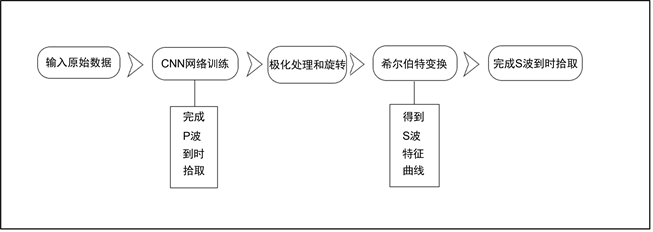Figure 1. Flow chart of seismic phase pickup model

3. 基于极化处理的S波特征提取

3.1. P波到时拾取网络的设计

CNN算法叫做卷积神经网络，是在人工神经网络的基础上发展而来的一种深度学习，是由多个卷积层、池化层及全连层构成的特征分类器，CNN是一种监督学习技术，可以直接应用于提取地震数据分类的幅度数据，相对于其他监督分类方法的主要优点是其空间感知和自动特征提取。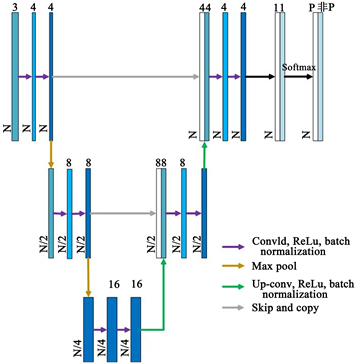Figure 2. CNN network structure diagram

3.2. 地震数据的极化处理和旋转

$\left(\begin{array}{c}{Z}^{\prime }\\ {Y}^{\prime }\\ {X}^{\prime }\end{array}\right)=\left(\begin{array}{c}\mathrm{sin}i-\mathrm{cos}\theta \mathrm{cos}i-\mathrm{sin}\theta \mathrm{cos}i\\ \mathrm{cos}i\mathrm{cos}\theta \mathrm{sin}i\mathrm{sin}\theta \mathrm{sin}i\\ 0-\mathrm{sin}\theta \mathrm{cos}\theta \end{array}\right)\left(\begin{array}{c}Z\\ Y\\ X\end{array}\right)$ (1)

3.3. 基于希尔伯特变换的S波特征提取

$X\left(j\right)=x\left(j\right)+iH\left\{x\left(j\right)\right\}$ (2)

$Y\left(j\right)=y\left(j\right)+iH\left\{y\left(j\right)\right\}$ (3)

$C{F}^{S}\left(j\right)={\lambda }_{1}{\left(j\right)}^{2}$ (4)

S波的特征曲线如图3所示。

4. 模型性能分析

4.1. 实际数据的应用效果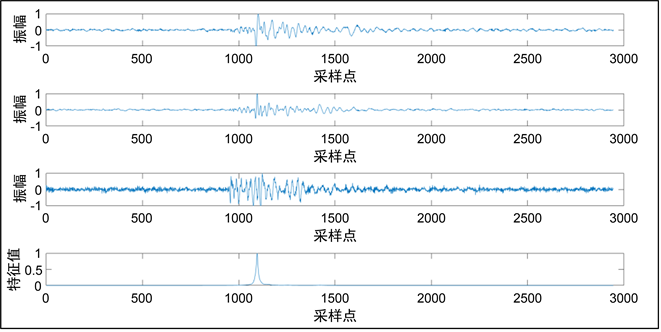Figure 3. Hilbert transform result. (a) Original x component seismic records; (b) Original y component seismic records; (c) Original z-component seismic records; (d) S Wave sign curve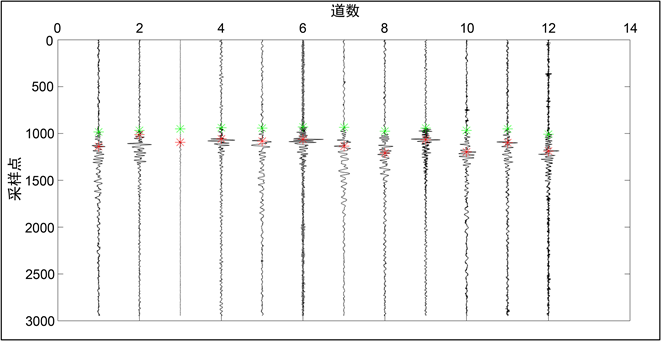Figure 4. Application results of seismic phase pickup model

4.2. 与传统短长时窗法的对比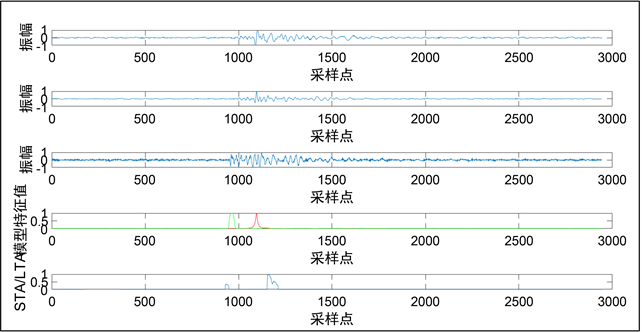Figure 5. Performance comparison of the two methods. (a) Original x component seismic records; (b) Original y component seismic records; (c) Original z-component seismic records; (d) The characteristic curve obtained by the model in this paper; (e) STA/LTA characteristic curve

4.3. 与一般CNN网络的对比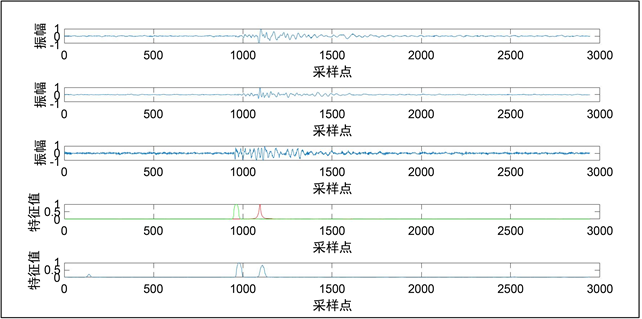Figure 6. Comparison of performance of two CNN networks. (a) Original x component seismic records; (b) Original y component seismic records; (c) Original z-component seismic records; (d) The characteristic curve obtained by the model in this paper; (e) Characteristic curve obtained by general CNN

5. 结论与讨论

 Wang, H., Li, M. and Shang, X.F. (2016) Developments on Micro-Seismic Data Processing. Journal of Natural Gas Science and Engineering, 32, 521-537.
https://doi.org/10.1016/j.jngse.2016.02.058

 Allen, R.V. (1978) Au-tomatic Earthquake Recognition and Timing from Single Traces. Bulletin of the Seismological Society of America, 68, 1521-1532.

 Tselentis, G., Matakis, N., Paraskevopoulos, P., Lois, A. and Sokos, E. (2012) Strategy for Automated Analysis of Passive Microseismic Data Based on Stransform, Otsu’s Thresholding, and Higher Order Statistics. Geo-physics, 7, KS43-KS54.
https://doi.org/10.1190/geo2011-0301.1

 Sheng, G.Q. (2019) Hydraulic Fracturing Microseismic First Arrival Picking Method Based on Non-Subsampled Shearlet Transform and Higher-Order-Statistics. Journal of Seismic Exploration, 28, 593-618.

 贾佳, 王夫运, 吴庆举. 机器学习在地震检测与震相识别的应用综述[J]. 地震工程学报, 2019, 41(6): 1419-1425.

 赵明, 陈石, Dave Yuen. 基于深度学习卷积神经网络的地震波形自动分类与识别[J]. 地球物理学报, 2019, 62(1): 374-382.

 Zheng, J., Lu, J., Peng, S. and Jiang, T. (2018) An Automatic Microseismic or Acoustic Emission Arrival Identification Scheme with Deep Recurrent Neural Networks. Geophysical Journal International, 212, 1389-1397.
https://doi.org/10.1093/gji/ggx487

 Ross, Z.E., Meier, M. and Hauksson, E. (2018) P-Wave Arrival Picking and First-Motion Polarity Determination with Deep Learning. Journal of Geophysical Research, 123, 5120-5129.
https://doi.org/10.1029/2017JB015251

 Zhu, W. and Beroza, G.C. (2018) Phasenet: A Deep-Neural-Network-Based Seismic Arrival Time Picking Method. Geophysical Journal International, 216, 261-273.
https://doi.org/10.1093/gji/ggy423

 Zheng, J., Shen, S., Jiang, T. and Zhu, W. (2019) Deep Neural Networks Design and Analysis for Automatic Phase Pickers from Three-Component Micro-Seismic Recordings. Geophysical Journal International, 220, 323-334.
https://doi.org/10.1093/gji/ggz441

 Grigoli, F., Cesca, S., et al. (2014) Automated Seismic Event Location by Waveform Coherence Analysis. Geophysical Journal International, 196, 1742-1753.
https://doi.org/10.1093/gji/ggt477

Top